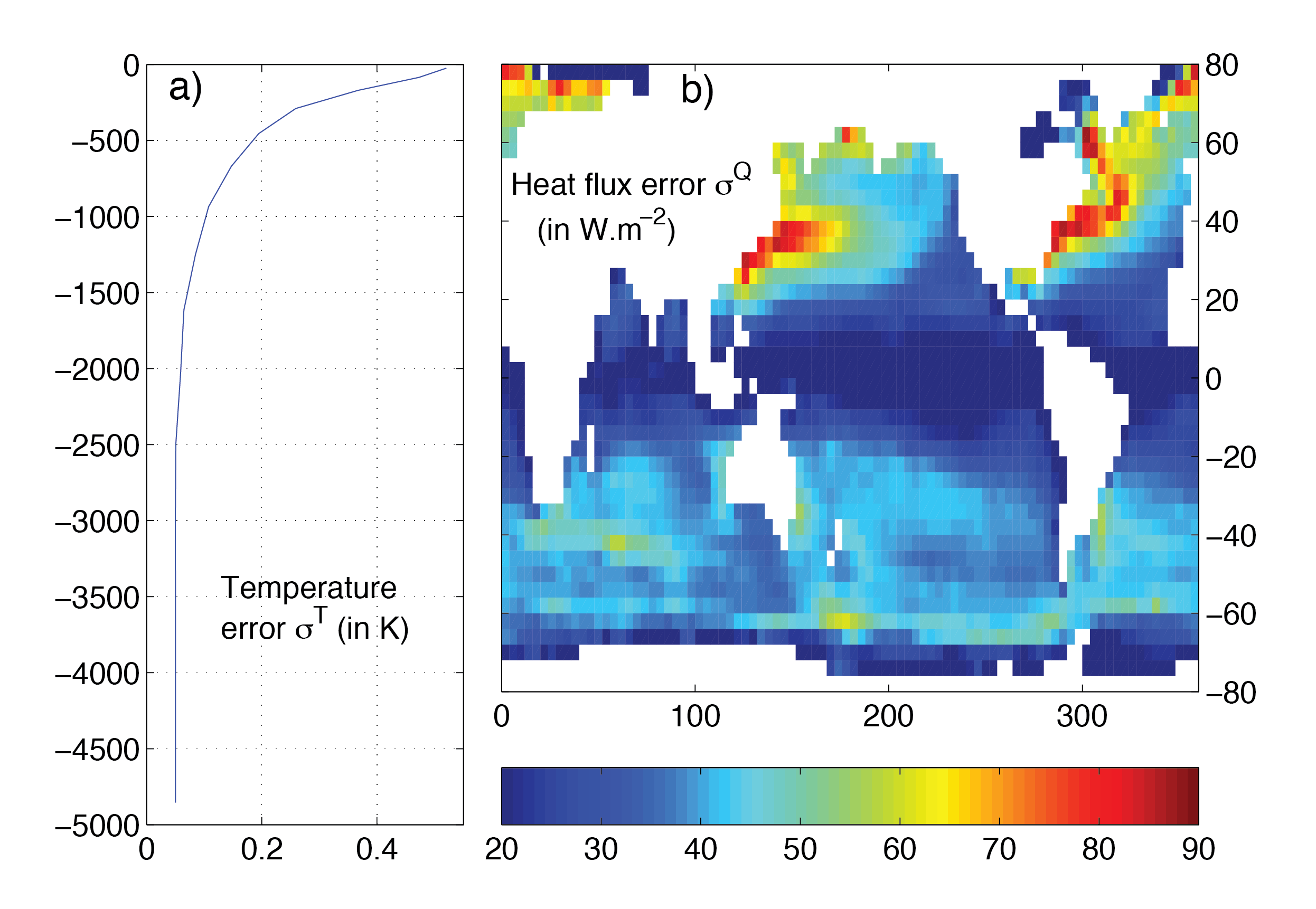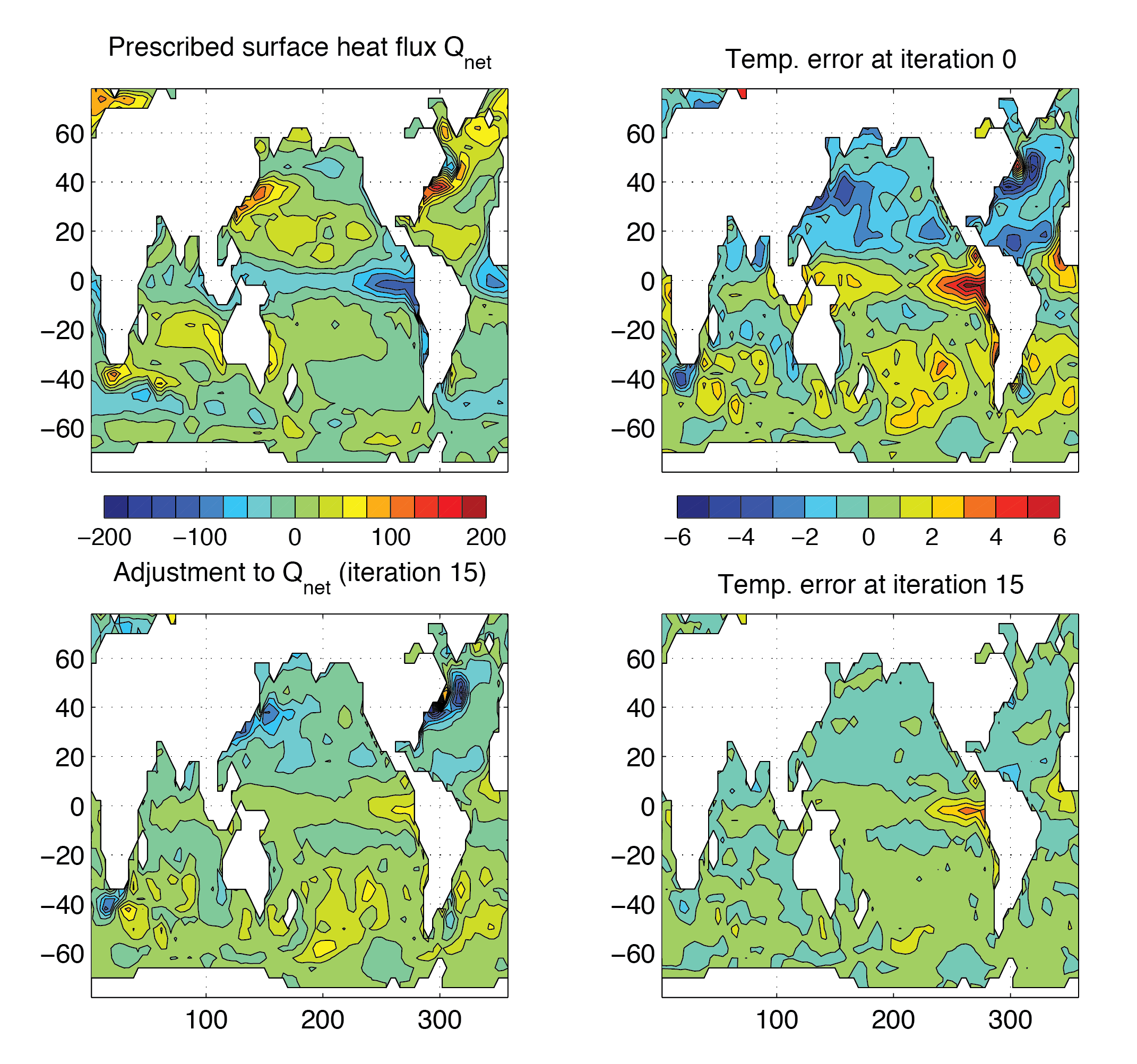# 4.11. Global Ocean State Estimation¶

(in directory: verification/tutorial_global_oce_optim/)

## 4.11.1. Overview¶

This experiment illustrates the optimization capacity of the MITgcm: here, a high level description.

In this tutorial, a very simple case is used to illustrate the optimization capacity of the MITgcm. Using an ocean configuration with realistic geography and bathymetry on a $$4\times4^\circ$$ spherical polar grid, we estimate a time-independent surface heat flux adjustment $$Q_\mathrm{netm}$$ that attempts to bring the model climatology into consistency with observations (Levitus and Boyer (1994a,b) [LB94a, LB94b]).

This adjustment $$Q_\mathrm{netm}$$ (a 2-D field only function of longitude and latitude) is the control variable of an optimization problem. It is inferred by an iterative procedure using an ‘adjoint technique’ and a least-squares method (see, for example, Stammer et al. (2002) [SWG+02] and Ferriera et a. (2005) [FMH05].

The ocean model is run forward in time and the quality of the solution is determined by a cost function, $$J_1$$, a measure of the departure of the model climatology from observations:

(4.58)$J_1=\frac{1}{N}\sum_{i=1}^N \left[ \frac{\overline{T}_i-\overline{T}_i^{lev}}{\sigma_i^T}\right]^2$

where $$\overline{T}_i$$ and $$\overline{T}_i^{lev}$$ are, respectively, the model and observed potential temperature at each grid point $$i$$. The differences are weighted by an a priori uncertainty $$\sigma_i^T$$ on observations (as provided by Levitus and Boyer (1994a) [LB94a]). The error $$\sigma_i^T$$ is only a function of depth and varies from 0.5 K at the surface to 0.05 K at the bottom of the ocean, mainly reflecting the decreasing temperature variance with depth (see Figure 4.47a). A value of $$J_1$$ of order 1 means that the model is, on average, within observational uncertainties.Figure 4.47 A priori errors on potential temperature (left, in oC) and surface heat flux (right, in W m-2) used to compute the cost terms $$J_1$$ and $$J_2$$, respectively.¶

The cost function also places constraints on the adjustment to insure it is “reasonable”, i.e., of order of the uncertainties on the observed surface heat flux:

$J_2 = \frac{1}{N} \sum_{i=1}^N \left[\frac{Q_\mathrm{netm}}{\sigma^Q_i} \right]^2$

where $$\sigma^Q_i$$ are the a priori errors on the observed heat flux as estimated by Stammer et al. (2002) [SWG+02] from 30% of local root-mean-square variability of the NCEP forcing field (see Figure 4.47b).

The total cost function is defined as $$J=\lambda_1 J_1+ \lambda_2 J_2$$ where $$\lambda_1$$ and $$\lambda_2$$ are weights controlling the relative contribution of the two components. The adjoint model then yields the sensitivities $$\partial J/\partial Q_\mathrm{netm}$$ of $$J$$ relative to the 2-D fields $$Q_\mathrm{netm}$$. Using a line-searching algorithm (Gilbert and Lemaréchal 1989 ), $$Q_\mathrm{netm}$$ is adjusted then in the sense to reduce $$J$$ — the procedure is repeated until convergence.

Figure 4.48 shows the results of such an optimization. The model is started from rest and from January-mean temperature and salinity initial conditions taken from the Levitus dataset. The experiment is run a year and the averaged temperature over the whole run (i.e., annual mean) is used in the cost function (4.58) to evaluate the model 1. Only the top 2 levels are used. The first guess $$Q_\mathrm{netm}$$ is chosen to be zero. The weights $$\lambda_1$$ and $$\lambda_2$$ are set to 1 and 2, respectively. The total cost function converges after 15 iterations, decreasing from 6.1 to 2.7 (the temperature contribution decreases from 6.1 to 1.8 while the heat flux one increases from 0 to 0.42). The right panels of Figure 4.48 illustrate the evolution of the temperature error at the surface from iteration 0 to iteration 15. Unsurprisingly, the largest errors at iteration 0 (up to 6 oC, top left panels) are found in the Western boundary currents. After optimization, the departure of the model temperature from observations is reduced to 1 oC or less almost everywhere except in the Pacific equatorial cold tongue. Comparison of the initial temperature error (top, right) and heat flux adjustment (bottom, left) shows that the system basically increased the heat flux out of the ocean where temperatures were too warm and vice-versa. Obviously, heat flux uncertainties are not solely responsible for temperature errors, and the heat flux adjustment partly compensates the poor representation of narrow currents (Western boundary currents, equatorial currents) at $$4\times4^\circ$$ resolution. This is allowed by the large a priori error on the heat flux Figure 4.47. The Pacific cold tongue is a counter example: there, heat fluxes uncertainties are fairly small (about 20 W m-2), and a large temperature errors remains after optimization.Figure 4.48 Initial annual mean surface heat flux (top right in W m-2) and adjustment obtained at iteration 15 (bottom right). Averaged difference between model and observed potential temperatures at the surface (in $$^\circ$$C) before optimization (iteration 0, top right) and after optimization (iteration 15, bottom right). Contour intervals for heat flux and temperature are 25 W m-2 and 1 oC, respectively. A positive flux is out of the ocean.¶

## 4.11.2. Implementation of the control variable and the cost function¶

One of the goals of this tutorial is to illustrate how to implement a new control variable. Most of this is fairly generic and is done in pkg/ctrl and pkg/cost. The modifications can be tracked by the CPP option ALLOW_HFLUXM_CONTROL or the comment cHFLUXM_CONTROL. The more specific modifications required for the experiment are found in verification/tutorial_global_oce_optim/code_ad. Here follows a brief description of the implementation.

### 4.11.2.1. The control variable¶

The adjustment $$Q_\mathrm{netm}$$ is activated by setting #define ALLOW_HFLUXM_CONTROL in code_ad/CTRL_OPTIONS.h.

It is first implemented as a “normal” forcing variable. It is defined in model/inc/FFIELDS.h, initialized to zero in model/src/ini_forcing.F, and then used in model/src/external_forcing_surf.F. $$Q_\mathrm{netm}$$ is made a control variable in pkg/ctrl by modifying the following subroutines:

• pkg/ctrl/ctrl_init.F where $$Q_\mathrm{netm}$$ is defined as the control variable number 24,

• pkg/ctrl/ctrl_pack.F which writes, at the end of each iteration, the sensitivity of the cost function $$\partial J/\partial Q_\mathrm{netm}$$ in to a file to be used by the line-search algorithm,

• pkg/ctrl/ctrl_unpack.F which reads, at the start of each iteration, the updated adjustment as provided by the line-search algorithm,

• pkg/ctrl/ctrl_map_forcing.F in which the updated adjustment is added to the first guess $$Q_\mathrm{netm}$$.

Note also some minor changes in pkg/ctrl/ctrl.h, pkg/ctrl/ctrl_readparms.F, and pkg/ctrl/ctrl_dummy.h (xx_hfluxm_file, fname_hfluxm, xx_hfluxm_dummy).

### 4.11.2.2. Cost functions¶

The cost functions are implemented using pkg/cost.

## 4.11.3. Code Configuration¶

The experiment files in verification/tutorial_global_oce_optim/code_ad/ and verification/tutorial_global_oce_optim/input_ad/ contain the code customizations and parameter settings. Most of them are identical to those used in the Global Ocean ( experiment verification/tutorial_global_oce_latlon/). Below, we describe some of the customizations required for this experiment.

### 4.11.3.2. Running-time customizations in input_ad¶

• input_ad/data: note the smaller cg2dTargetResidual than in the forward-only experiment,

• input_ad/data.optim specifies the iteration number,

• input_ad/data.ctrl is used, in particular, to specify the name of the sensitivity and adjustment files associated to a control variable,

• input_ad/data.cost: parameters of the cost functions, in particular lastinterval specifies the length of time-averaging for the model temperature to be used in the cost function (4.58),

• input_ad/data.pkg: note that the Gradient Check package is turned on by default (useGrdchk =.TRUE.),

• Err_hflux.bin and Err_levitus_15layer.bin are the files containing the heat flux and potential temperature uncertainties, respectively.

## 4.11.4. Compiling¶

The optimization experiment requires two executables: 1) the MITgcm and its adjoint (mitgcmuv_ad) and 2) the line-search algorithm (optim.x).

### 4.11.4.1. Compilation of MITgcm and its adjoint: mitcgmuv_ad¶

Before compiling, first note that in the directory code_ad, two files must be updated:

Then, in the directory build, type:

% ../../../tools/genmake2 -mods=../code_ad -adof=../code_ad/ad_optfile.local
% make depend


to generate the MITgcm executable mitgcmuv_ad.

### 4.11.4.2. Compilation of the line-search algorithm: optim.x¶

This is done from the directories lsopt/ and optim/ (found in the top MITgcm directory). In lsopt/, unzip the blash1 library adapted to your platform (see lsopt/README), and change the Makefile accordingly. Compile with:

% make all


(more details in lsopt/lsopt_doc.txt)

In optim/, the path of the directory where mitgcm_ad was compiled must be specified in the Makefile in the variable INCLUDEDIRS. The file name of the control variable (here, xx_hfluxm_file) must be added to the namelist read by optim/optim_numbmod.F. Then use

% make depend


and

% make


to generate the line-search executable optim.x.

## 4.11.5. Running the estimation¶

Make a new subdirectory input_ad/OPTIM. Copy the mitgcmuv_ad executable to input_ad and optim.x to this subdirectory. cd into input_ad/. The first iteration is somewhat particular and is best done “by hand” while the following iterations can be run automatically (see below). Check that the iteration number is set to 0 in input_ad/data.optim and run MITgcm:

% ./mitgcmuv_ad


The output files adxx_hfluxm.0000000000.* and xx_hfluxm.0000000000.* contain the sensitivity of the cost function to $$Q_\mathrm{netm}$$ and the adjustment to $$Q_\mathrm{netm}$$ (zero at the first iteration), respectively. Two other files called costhflux_tut_MITgcm.opt0000 and ctrlhflux_tut_MITgcm.opt0000 are also generated. They essentially contain the same information as the adxx_.hfluxm* and xx_hfluxm* files, but in a compressed format. These two files are the only ones involved in the communication between the adjoint model mitgcmuv_ad and the line-search algorithm optim.x. Only at the first iteration, are they both generated by mitgcmuv_ad. Subsequently, costhflux_tut_MITgcm.opt $$n$$ is an output of the adjoint model at iteration $$n$$ and an input of the line-search. The latter returns an updated adjustment in ctrlhflux_tut_MITgcm.opt $$n+1$$ to be used as an input of the adjoint model at iteration $$n+1$$.

At the first iteration, move costhflux_tut_MITgcm.opt0000 and ctrlhflux_tut_MITgcm.opt0000 to input_ad/OPTIM, move into this directory and link input_ad/data.optim and input_ad/data.ctrl locally:

% cd OPTIM/
% ln -s ../data.optim .
% ln -s ../data.ctrl .


The target cost function fmin needs to be specified in input_ad/data.optim: as a rule of thumb, it should be about 0.95-0.90 times the value of the cost function at the first iteration. This value is only used at the first iteration and does not need to be updated afterward. However, it implicitly specifies the “pace” at which the cost function is going down (if you are lucky and it does indeed diminish!).

Once this is done, run the line-search algorithm:

% ./optim.x


which computes the updated adjustment for iteration 1, ctrlhflux_tut_MITgcm.opt0001.

The following iterations can be executed automatically using the shell script input_ad/cycsh. This script will take care of changing the iteration numbers in input_ad/data.optim, launch the adjoint model, clean and store the outputs, move the costhflux* and ctrlhflux* files, and run the line-search algorithm. Edit input_ad/cycsh to specify the prefix of the directories used to store the outputs and the maximum number of iteration.

1

Because of the daily automatic testing, the experiment as found in the repository is set-up with a very small number of time-steps. To reproduce the results shown here, one needs to set nTimeSteps = 360 and lastinterval =31104000 (both corresponding to a year, see Section 4.11.3.2 for further details).